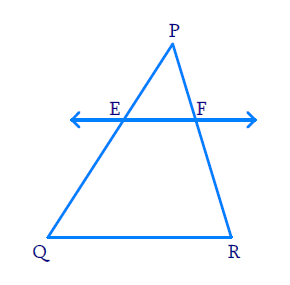# Ex.6.2 Q2 Triangles Solution - NCERT Maths Class 10

Go back to  'Ex.6.2'

## Question

$$E$$ and $$F$$ are points on the sides $$PQ$$ and $$PR$$ respectively of a $$\Delta \mathrm{PQR}$$. For each of the following cases, state whether $$EF || QR:$$

(i)

$$PE = 3.9\,\rm{cm},\; EQ = 3\,\rm{cm},\; PF = 3.6 \,\rm{cm}$$ and $$FR = 2.4\,\rm{cm}$$

(ii)

$$PE = 4\,\rm{cm},\; QE = 4.5\,\rm{cm},\; PF = 8\,\rm{cm}$$ and $$RF = 9\,\rm{cm}$$

(iii)

\begin{align}PQ &= 1.28\,\rm{cm},\;\\ PR &= 2.56 \,\rm{cm}, \\\;PE &= 0.18\,\rm{cm} \\PF &= 0.36\,\rm{cm}\end{align}

Video Solution
Triangles
Ex 6.2 | Question 2

## Text Solution

(i) Reasoning:

As we know that a line divides any two sides of a triangle in the same ratio, then the line is parallel to the third side (converse of $$BPT$$)

Steps:Here,

\begin{align} \frac{PE}{EQ}&=\frac{3.9}{3} =1.3\,\text{cm} \\\\ & \rm{and}\\\\ \frac{PF}{FR}&=\frac{3.6}{2.4}= 1.5 \end{align}

Hence,

\begin{align} \frac {{PE}}{EQ} \not= \frac {{PF}}{FR} \end{align}

According to converse of $$BPT,\;EF$$ is not parallel to $$QR$$.

(ii) Reasoning:

As we know that a line divides any two sides of a triangle in the same ratio, then the line is parallel to the third side(converse of $$BPT$$)

Steps:

Here,

\begin{align} \frac{{PE}}{EQ}= \frac{{4}}{4.5}=\frac{{8}}{9} \end{align}

and

\begin{align} \frac{{PF}}{FR}= \frac{{8}}{9} \end{align}

Hence,

\begin{align} \frac{{PE}}{EQ}=\frac{{PF}}{FR} \end{align}

According to converse of $$BPT$$$$EF \parallel QR$$

(iii) Reasoning:

As we know that a line divides any two sides of a triangle in the same ratio, then the line is parallel to the third side(converse of $$BPT$$)

Steps:

Here,

$$PQ=1.28 \,\rm{cm}$$  and $$PE=0.18\,\rm{cm}$$ 

\begin{align} {EQ} &={PQ}-{PE} \\ &=(1.28-0.18)\,\rm{cm} \\ &=1.10\, \mathrm{cm} \end{align}

$$PR=2.56\,\rm{cm}$$ and $$PF=0.36\,\rm{cm}$$

\begin{align} {FR} &={PR}-{PF}\\ &=(2.56-0.36)\,\rm{}cm \\ &=2.20\; \mathrm{cm} \end{align}

Now,

\begin{align} \frac{PE}{EQ}&=\frac{0.18\text{cm}}{1.10\text{cm}} =\frac{18}{110} =\frac{9}{55} \\ \frac{PF}{FR} &=\frac{0.36\text{cm}}{2.20\text{cm}} =\frac{36}{220}=\frac{9}{55} \end{align}

\begin{align}\Rightarrow\frac{PE}{EQ}=\frac{PF}{FR} \end{align}

According to converse of $$BPT,\;EF\parallel QR$$ 

Learn from the best math teachers and top your exams

• Live one on one classroom and doubt clearing
• Practice worksheets in and after class for conceptual clarity
• Personalized curriculum to keep up with school